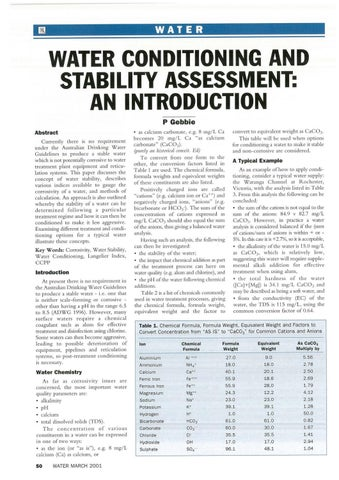m

WATER

WATER CONDITIONING AND STABILITY ASSESSMENT: AN INTRODUCTION P Gebbie Abstract Currently there is no requirement under the Australian Drinking W ater Guidelines to produ ce a stable water w hich is not potentially corrosive to water treatment plant equipment and reticulation systems. This paper disc usses the concept of w ater stability, d esc ribes various indices available to ga uge the corrosivity o f a water, and m etho ds o f calculation. An approach is also outlined w hereby the stability of a ,Nater can be d e termin ed fo ll ow ing a pa rtic ular treatment regime and how it can then be conditioned to make it less aggressive . Examining different treatm ent and conditioning options for a typi ca l w ater illustrate th ese concepts. Key Words: Corrosivity, Water Stability, W ater C onditioning, Langelier Ind ex, CCPP

Introduction At present there is no requirement in the Australian Drinkin g Water Guidelin es to produce a stable water - i.e. one that is neither scale-fo rming or corrosive other than having a pH in the range 6.5 to 8.5 (ADWG 1996). H owever, ma ny surfac e waters require a c hemi cal co agulant such as alum for effective treatment and disinfection using chlorine. Som e waters can then becom e aggressive, leadin g to possible deterio ratio n of equipment, pip elines and reticulation system s, so post- treatment conditio ning 1s necessa ry.

Water Chemistry As far as co rros ivity issu es are concerned, th e most impo rtant water quality param eters are: • alka linity • pH • calcium • total dissolved solids (TDS). Th e c o nce ntrat i on o f v ario u s constitu ents in a water can be expressed in one o f tw o ways: • as the ion (or "as is"), e .g. 8 mg/L calci um (C a) as calcium, or 50

WATER MARCH 2001

• as calcium carbonate, e.g. 8 m g/L Ca becomes 20 m g/ L Ca "as calcium carbonate" (CaC O 3) . (purely an historical conceit. Ed) T o con vert from one fo rm to the other, the conversio n factors listed in T able 1 are used . T he chemical for mula, formula we ights and equi valen t weights of these constituen ts are also listed. Positively charged io ns are called "catio ns" (e .g. calcium ion or c a++) and negati vely charged ions, "anio ns" (e.g. bicarbo nate or H C O 3 -) . T he sum of the concentration of cations expressed as m g/L C aCO 3 should also equal the sum of the anions, thus giving a balanced water analysis. Having such an analysis, th e fo llowing can then be investigated: • the stability of the wa ter; • the impact that chemical addition as part of th e treatment process can have o n water quality (e.g. alum and chlorine), and • the pH of the water fo llowing chemical add itions. T able 2 is a list of chemicals corrunonly used in water treatment processes, giving th e chemical fo rmula , formula w eight, equivalent weight and the factor to

con vert to equivalen t weight as C aCO 3. T his table w ill be used wh en optio ns for con ditionin g a water to make it stable and no n-corrosive are considered.

A Typical Example As an exa mple of how to apply conditioning, consider a typical w ater suppl y: the W aranga C hannel at R ochester, Victo ria, w ith the analysis listed in Table 3. From this analysis the following can be co ncluded: • the sum of the cations is not equal to the sum of the anions: 84.9 v 82.7 mg/ L CaCO 3 . H owever, in practice a water analysis is considered balanced if the (sum of cations/sum of anions is within + or 5%. In this case it is +2.7%, so it is acceptable, • the alkalini ty of the water is 15 .0 m g/L as CaCO 3, which is relatively low, suggesting this water will require supplem e ntal alkali additi o n for effe cti ve trea tment w hen using alum , • th e total hardn ess of the wate r ([Ca]+ (Mg]) is 34.1 m g/L C aCO3 and may be described as being a soft water, and • from the conductivity (E C) of the w ater, the TDS is 115 mg/ L , using the common conve rsion factor of 0.64.

Table 1. Chemical Formula, Formula Weight, Equiva lent Weight and Factors to Convert Concentration from "AS IS" to "CaC0 3 " for Common Cations and Anions Ion

Chemical Formula

Formula Weight

Equivalent Weight

As CaCO3 Multiply by

Aluminium

Al +++

27.0

9.0

5.56

Ammoni um

18.0

18.0

2.78

Calcium

NH4+ ca++

40.1

20.1

2.50

Ferric Iron

Fe+++

55.9

18.6

2.69

Ferrous Iron

Fe++

55.9

28.0

1.79

Magnesium

Mg++

24.3

12.2

4.12

Sodi um

Na+

23.0

23.0

2.18

Potassium

K+

39.1

39.1

1.28

Hydrogen

H+

1.0

1.0

50.0

Bicarbonate

HC0 3· C03··

61.0

61.0

0.82

Carbonate

60.0

30.0

1.67

Chloride

Cl·

35.5

35.5

1.41

Hydroxide

OH·

17.0

17.0

2.94

Sulphate

S04··

96 .1

48.1

1.04